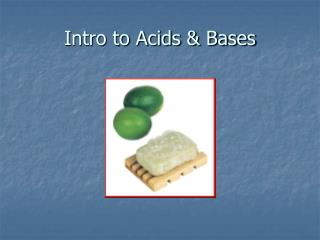DownloadDownload PresentationIntro to Acids & Bases

# Intro to Acids & Bases

Télécharger la présentation## Intro to Acids & Bases

- - - - - - - - - - - - - - - - - - - - - - - - - - - E N D - - - - - - - - - - - - - - - - - - - - - - - - - - -
##### Presentation Transcript

1. Intro to Acids & Bases

2. Acids Taste sour Reacts with metals Turns litmus red Conducts electricity Base Taste bitter Slippery Turns litmus blue Conducts electricity Properties of Acids & Bases

3. Ions in Solution • Acidic solutions – contain more H+ than OH- • Basic solutions – contain more OH- than H+ • Neutral solutions – contain equal amounts of H+ and OH-

4. Autoionization of Water • H2O + H2O  H3O+ + OH- • Water is the usual solvent for acids and bases • It produces equal numbers of H3O+ and OH-

5. Arrhenius Model of Acids & Bases • Acid: a substance that contains H and ionized to produce H+ when dissolved in water. • Base: a substance that contains OH and ionizes to produce OH- when dissolved in water

6. Arrhenius Model of Acids & Bases • HCl  H+ + Cl- • HCl contains H and ionizes to form H+ • This could be an Arrhenius acid • NaOH  Na+ + OH- • NaOH contains OH and ionized to form OH- • This could be considered an Arrhenius base

7. Arrhenius Model of Acids & Bases • Although the Arrhenius model is useful in describing many acids and bases, it does not describe them all • For example NH3 contains no OH- ions, but it is a base • A model to describe all bases is needed

8. Bronsted-Lowry Model • Acid: proton donor • Base: proton acceptor • HX + H20  H3O+ + X- • HX donates an H+ to the water molecule • The water takes the H and is there for considered the base

9. Bronsted-Lowry Model • Conjugate acid – the species produced when a base accepts the H+ ion from the acid • Conjugate base – the species produced when the acid gives up its H+

10. Conjugate Acids & Conjugate Bases • Identify the acid, base, conjugate acid, and conjugate base of the following reaction… • HX + H20  H3O+ + X- • A B CA CB • Every Bronsted-Lowry interaction involves conjugate acid base pairs

11. Conjugate Acids & Conjugate Bases • Identify the acid, base, conjugate acid, and conjugate base of the following reaction… • NH3 + H20  NH4+ + OH- • B A CA CB

12. Conjugate Acids & Conjugate Bases • What did you notice that was different about the previous two reactions? • Water was an acid in one and a base in the other • Amphoteric – substance that can act as either an acid or a base

13. Conjugate Acids & Conjugate Bases • Identify the acid, base, conjugate acid, and conjugate base of the following reactions… • NH4+ + OH- NH3 + H20 • A B CB CA • HBr + H2O  H3O+ + Br - • A B CA CB

14. Neutralization Reactions • Neutralization reactions – acid + base  a salt + water • Neutralization reactions are just a special type of double replacement reactions

15. Neutralization Reactions • Write the equations for the following neutralization reactions • Acetic acid and ammonium hydroxide • HCH3COO + NH4OH  HOH + NH4CH3COO • Nitric acid and cesium hydroxide • HNO3 + CsOH  HOH + CsNO3

16. The pH Scale • [H+] is often expressed in very small numbers. Chemists needed an easier way to express [H+] ions • pH is a mathematical scale in which the concentration of hydronium ions in a solution is expressed as a number from 0 to 14. • pH = -log[H+]

17. Interpreting the pH Scale • pH of 7 is neutral. A pH less than 7 is acidic, and a pH greater than 7 is basic.

18. pH Calculations • pH = -log[H+] • pOH = -log[OH-] • pH + pOH = 14

19. Calculating pH • What is the pH of a 0.5M HCl solution? • 0.5 mol HCl x 1 mol H+ = 0.5M H+ L 1 mol HCl • pH = -log[H+] • pH = -log[0.5] • pH = 0.3

20. Calculating pH • Calculate the pH of a 0.0057M HBr solution. • 2.2

21. Calculating pH • What if we have a base? • What is the pH of a 0.05M NaOH solution? • 0.05 mol NaOH x 1 mol OH- = 0.05M OH- L 1 mol NaOH • pOH = -log[OH-] • pOH = -log[0.05] • pOH = 1.3 • pH + pOH = 14 • pH = 12.7

22. Calculating pH • Calculate the pH of a 0.000089M KOH solution. • 9.95

23. Acid/Base Strength • The strength of an acid or a base tells you the degree of ionization • Strong acids & bases break down into many ions • Weak acids & bases break down into just a few ions

24. Acid/Base Strength

25. Acid/Base Strength